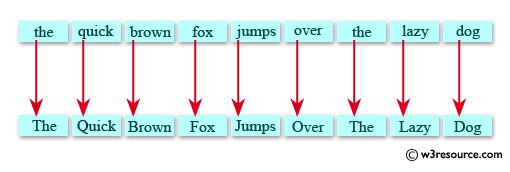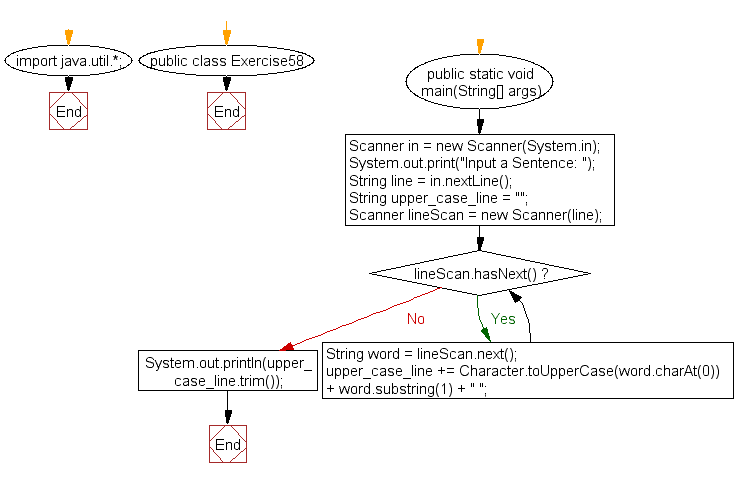﻿ Java: Capitalize the first letter of each word in a sentence# Java Exercises: Capitalize the first letter of each word in a sentence

## Java Basic: Exercise-58 with Solution

Write a Java program to capitalize the first letter of each word in a sentence.

Pictorial Presentation: Capitalize the first letter of each word in a sentenceSample Solution:

Java Code:

``````import java.util.*;
public class Exercise58 {
public static void main(String[] args){
Scanner in = new Scanner(System.in);
System.out.print("Input a Sentence: ");
String line = in.nextLine();
String upper_case_line = "";
Scanner lineScan = new Scanner(line);
while(lineScan.hasNext()) {
String word = lineScan.next();
upper_case_line += Character.toUpperCase(word.charAt(0)) + word.substring(1) + " ";
}
System.out.println(upper_case_line.trim());
}
}
```
```

Sample Output:

```Input a Sentence: the quick brown fox jumps over the lazy dog.
The Quick Brown Fox Jumps Over The Lazy Dog.
```

Flowchart:Java Code Editor:

What is the difficulty level of this exercise?

Test your Programming skills with w3resource's quiz.

﻿

## Java: Tips of the Day

countOccurrences

Counts the occurrences of a value in an array.

Use Arrays.stream().filter().count() to count total number of values that equals the specified value.

```public static long countOccurrences(int[] numbers, int value) {
return Arrays.stream(numbers)
.filter(number -> number == value)
.count();
}
```

Ref: https://bit.ly/3kCAgLb